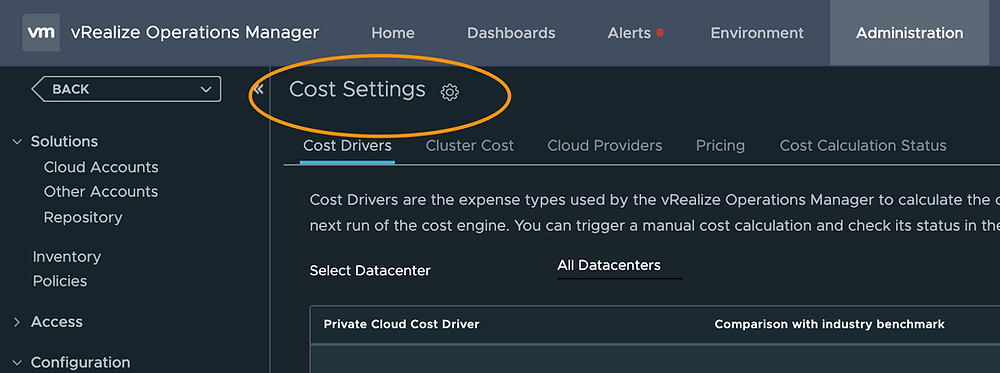Search
•Brock Peterson

# VM Cost with VMware vRealize Operations

Updated: May 9

You want to know how much a certain VM costs? No problem, vRealize Operations (vROps) has you covered. Specifically, it'll tell you how much you're paying for CPU, Memory, and Disk on that VM. How are these calculations made? Roughly like this:

1. Input ESXi Host Costs

2. Configure Cluster Costs

3. Determine CPU and Memory Base Rates

4. Calculate VM Costs based on CPU, Memory, and Disk Used

First, tell vROps which currency you'd like to use, enable cost calculation, and input the cost of your servers. We covered these item in a previous blog:

vROps provides great detail around ESXi Host costing: servers, storage, license costs, application costs, maintenance, labor, network, facilities, and miscellaneous costs you might want to add.This is where you will get your Disk base rate. The industry benchmark is \$.10/GB/month, but you can adjust as necessary here.vROps also provides the ability to depreciate your hardware from two to five years. To adjust the depreciation schedule go here:After clicking the gears icon you'll be presented with two options:The default is linear (straight line) depreciation over five years. If an ESXi Host cost \$10,000 to buy and is put on a five year depreciation schedule, it will depreciate \$2000 per year until it's are worth \$0 at the end of five years. You can adjust the number of years down to as low as two and select the Max of Double or Straight line depreciation as well. Both methods are detailed here:

Once you've configured your ESXi Host costs and depreciation schedule you are ready to configure Cluster Costs via the Cluster Cost tab within Cost Settings.vROps provides two ways to do this, click CHANGE to see them.The default method is Cluster Usable Capacity after HA and Buffer. This is the total capacity left after resources have been allocated for HA and Buffer. An example using round numbers might look like this:

ESXi Host 1: 100 GHz CPU, 200 GB Memory, Cost \$10,000

ESXi Host 2: 100 GHz CPU, 200 GB Memory, Cost \$10,000

ESXi Host 3: 100 GHz CPU, 200 GB Memory, Cost \$10,000

If Cluster 1 is comprised of ESXi Hosts 1-3, we have the following:

Cluster 1: 300 GHz CPU, 600GB Memory, Cost \$30,000

If we remove 30 GHz CPU and 60GB RAM for HA and Buffer, we have 270GHz CPU and 540GB Memory remaining in Cluster 1. These are the totals used to determine CPU and Memory base rates, which are used to determine VM costs.

vROps determines base rates as follows: https://docs.vmware.com/en/vRealize-Operations-Manager/7.5/com.vmware.vcom.config.doc/GUID-6E52B303-A409-48D8-BFEA-2FB59C0CCFB6.html

ESXi Host cost is split into CPU and Memory costs based on the industry standard cost ratios for different server models. Disk cost is determined by the Storage Cost Driver you configured originally, the industry standard for Disk is \$.10/GB/month.

CPU base rate is computed by dividing the CPU cost of the cluster by the CPU capacity of the cluster. In our example, assuming we're using Cluster Usable Capacity after HA and Buffer we have the following:

Cluster 1: 270 GHz CPU, 540GB Memory, Cost \$30,000

Let's assume a 50/50 split between CPU and Memory costs (the vROps costing engine will do this for you). This leaves us with:

Cluster 1: 270GHz CPU for \$15,000 and 540GB Memory for \$15,000

CPU base rate = \$15,000 / 270 GHz and Memory base rate = \$15,000 / 540GB, so

CPU base rate = \$55.55/GHz and a Memory base rate = \$27.78/GB

The second method of Cluster Cost Calculation is Cluster Actual Utilization. The same method as above is used to calculate base rates in this case.We now have base rates for CPU, Memory, and Disk. Let's apply these rates to determine VM costs. The vROps costing engine uses average utilization rates to determine cost. For example, VMs in Cluster 1 will have the following base rates:

CPU: \$55.55/GHz

Memory: \$27.78/GB

Disk: \$.10/GB

The vROps costing engine calculates VM cost based on average daily usage of each resource. Let's consider a VM with the following usage metrics:

CPU: Usage average Daily is .1GHz

Memory: Consumed average Daily is .3GB

Disk: Virtual Machine used is 20GB, which is roughly .66GB daily (assuming a 30 day month)

In this case we will have the following daily costs:

CPU: \$5.55/day (\$55.55 x .1)

Memory: \$8.34/day (\$27.78 x .3)

Disk: \$.07/day (\$.10 x .66)

Total: \$13.96/day

vROps will make all of these calculations for you based on your Cost Settings. I've created a Cost Detail dashboard to show detailed costs metrics at each layer of a vSphere Environment, it's available out on VMware {code}: https://code.vmware.com/samples?id=7600